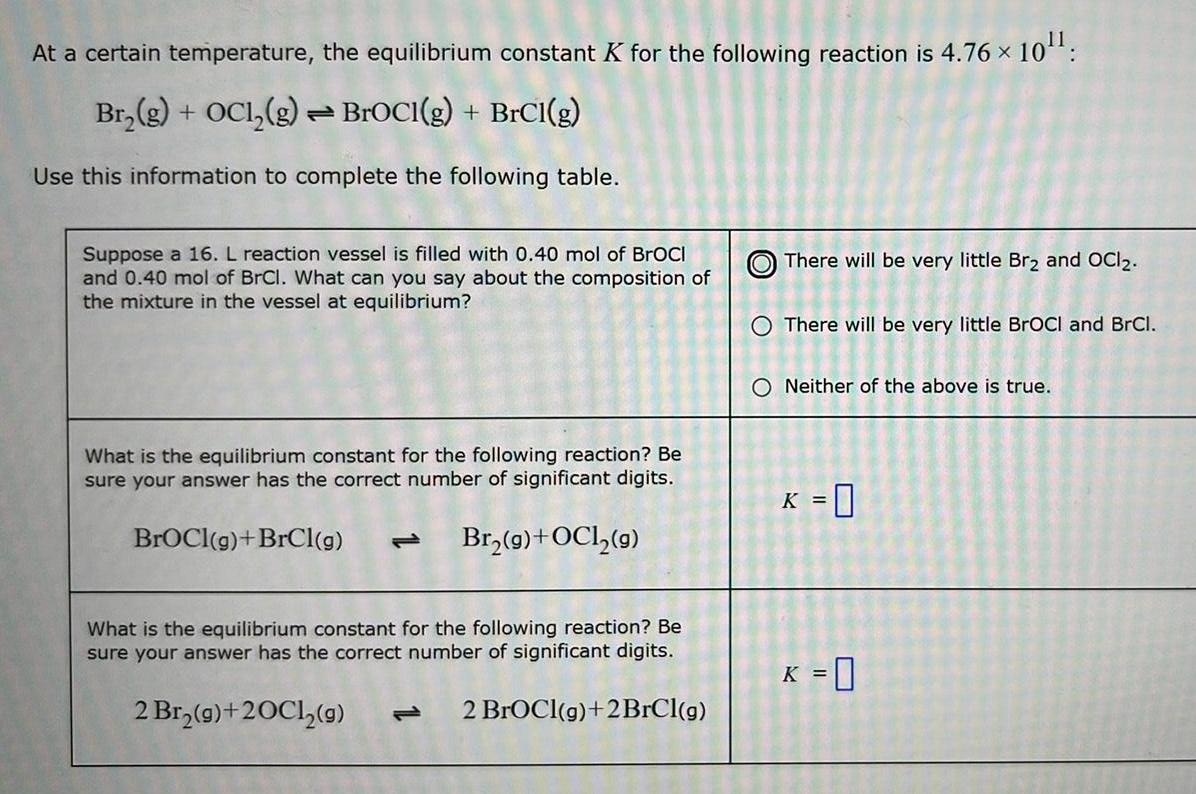Question:

# At a certain temperature, the equilibrium constant K for the

Last updated: 11/1/2022At a certain temperature, the equilibrium constant K for the following reaction is 4.76 × × 10¹¹: Br₂(g) + OC1₂(g) → BrOCI(g) + BrCl(g) Use this information to complete the following table. Suppose a 16. L reaction vessel is filled with 0.40 mol of BrOCI and 0.40 mol of BrCl. What can you say about the composition of the mixture in the vessel at equilibrium? What is the equilibrium constant for the following reaction? Be sure your answer has the correct number of significant digits. BrOCI(g) +BrCl(g) Br₂(g) + OCl₂(g) What is the equilibrium constant for the following reaction? Be sure your answer has the correct number of significant digits. 2 Br₂(g) +20C1₂(g) 2 BrOCI(g) +2BrCl(g) There will be very little Br₂ and OCI2. O There will be very little BrOCI and BrCl. O Neither of the above is true. K = 0 K = 0